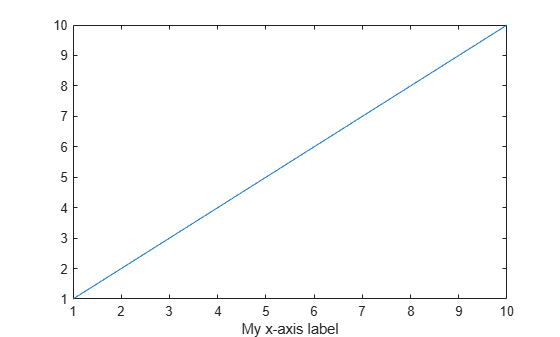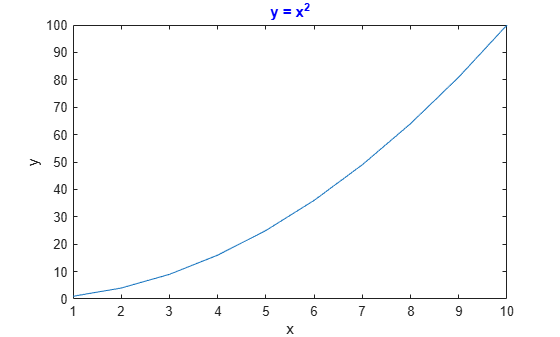findall

Find all graphics objects

Description

example

h = findall(objhandles) returns the graphics objects in objhandles and all of their descendants. Unlike the findobj function, findall returns objects even if their HandleVisibility property is set to 'off'.

example

h = findall(objhandles,prop1,value1,...,propN,valueN) returns the handles of all objects in the hierarchy that have the specified properties set to the specified values. For example, h = findall(gcf,'Type','text',Color','r') returns all text objects in the current figure that have a red color.

Examples

collapse all

Create three figures. Set the HandleVisibility property of the last figure to 'off'.

f1 = figure;
f2 = figure;
f3 = figure('HandleVisibility','off');

Display the number of visible or hidden handles in the graphics object hierarchy. Your result might be different from the result shown.

h1 = findall(groot);
disp(numel(h1))
4

Find all visible or hidden figures.

h2 = findall(groot,'Type','figure')
h2 =
3x1 Figure array:

Figure    (3)
Figure    (2)
Figure    (1)

If you try to find the figures using the findobj function, MATLAB® returns only f1 and f2.

h3 = findobj('Type','figure')
h3 =
2x1 Figure array:

Figure    (2)
Figure    (1)

Text objects within a figure have hidden handles. Use findall to return these hidden handles.

Create a figure with a plot. Then, create a label for the x-axis.

plot(1:10)
txt = xlabel('My x-axis label');Verify that the HandleVisibility property on txt is set to 'off'.

txt.HandleVisibility
ans =
'off'

Use findall to return the Text object for the x-axis label.

h1 = findall(gcf,'Type','text')
h1 =
Text (My x-axis label) with properties:

String: 'My x-axis label'
FontSize: 11
FontWeight: 'normal'
FontName: 'Helvetica'
Color: [0.1500 0.1500 0.1500]
HorizontalAlignment: 'center'
Position: [5.0000 0.3947 -1]
Units: 'data'

Show all properties

Because the Text object is hidden, you cannot find it using the findobj function.

h2 = findobj(gcf,'Type','text')
h2 =
0x0 empty GraphicsPlaceholder array.

Use findall to return all Text objects or Text objects with specific properties.

Create a figure with a plot. Then, label the axes and add a title to the axes. Set the color of the title to blue.

plot((1:10).^2)
xlabel('x')
ylabel('y')
title('y = x^2','Color','b')Return all Text objects in the current figure.

h1 = findall(gcf,'Type','text')
h1 =
3x1 Text array:

Text    (y = x^2)
Text    (x)
Text    (y)

Now, return all blue Text objects.

h2 = findall(gcf,'Type','text','Color','b')
h2 =
Text (y = x^2) with properties:

String: 'y = x^2'
FontSize: 11
FontWeight: 'bold'
FontName: 'Helvetica'
Color: [0 0 1]
HorizontalAlignment: 'center'
Position: [5.0000 100.7725 1.4211e-14]
Units: 'data'

Show all properties

Input Arguments

collapse all

Objects to search from, specified as an array of graphics objects. findall searches the objects in the input array objhandles and all of their descendants in the graphics object hierarchy.

Example: h = findall(groot) returns all visible and hidden handles in the graphics object hierarchy.

Property name, specified as a character vector or string scalar. For more information, see Graphics Object Properties.

Example: h = findall(gcf,'Type','text') returns all objects in the current figure whose Type property is set to 'text'.

Property value, specified as a scalar or array.

Tips

• To customize your search with findall, you can use objhandles followed by the input combinations from the findobj function. For example:

h = findall(groot,prop1,value1,'-not',prop2,value2,'-property',prop3)